/

### Function Description

Returns the weighted (Pearson) correlation between two series.

The weighted correlation for two series, 1 and 2, given weights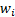(for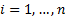), is calculated as: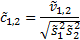where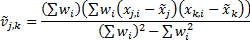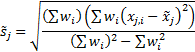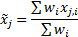See Weighted Moments and Cumulants for further details and explanation of terms in this formula. If the weights are equal then returns the same as MnCorrelation.

Contents | Prev | Next